# Random Blaschke products and Mathematica binding

A Blaschke product is a function that is the product of Blaschke factors, functions of the form

b(z; a) = |a|  (a – z) / a (1 – a*z)

where the complex number a lies inside the unit circle and a* is the complex conjugate of a.

I wanted to plot Blaschke products with random values of a using Mathematica, and I ran into a couple items of interest.

First, although Mathematica has a function RandomComplex for returning complex numbers chosen by a pseudorandom number generator, this function selects complex numbers uniformly over a rectangle and I wanted to select uniformly over a disk. This is easy enough to get around. I wrote my own function by selecting a magnitude and phase at random.

    rc[] := Sqrt[RandomReal[]] Exp[-2 Pi I RandomReal[]]

Now if I reuse the definition of a Blaschke factor from an earlier post

    b[z_, a_] := (Abs[a]/a) (a - z)/(1 - Conjugate[a] z)

I can define a product of two random Blaschke factors as follows.

    f[z_] := b[z, rc[]]  b[z, rc[]]

However, this may not do what you expect. If you plot the function twice, you’ll get different results! It’s a matter of binding order. At what point in the process are the two random values of a chosen and fixed? The answer is some time between the definition of f and executing the plotting function. If the plotting function generated new values of a every time it needed to evaluate f the result would be a hot mess.

On the other hand, if we leave out the colon then the function f behaves as expected.

    f[z_] = b[z, rc[]]  b[z, rc[]]

Now random values are generated by each call to rc, and these values are frozen in the definition of f.

Here’s a Blaschke product with 10 random parameters.

    f[z_] = Product[b[z, rc[]], {i, 1, 10}]

The code

    ComplexPlot[f[z], {z, -1 - I, 1 + I}]

produces the following plot.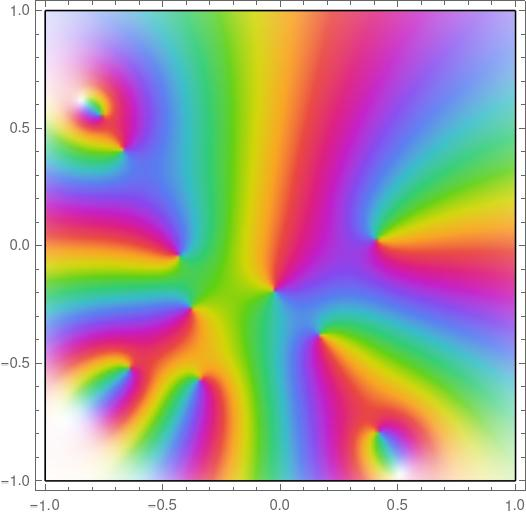If I plot this function again, say changing the plot range, I’ll plot the same function. But if I execute the line of code defining f again, and call ComplexPlot again, I’ll generate a new function.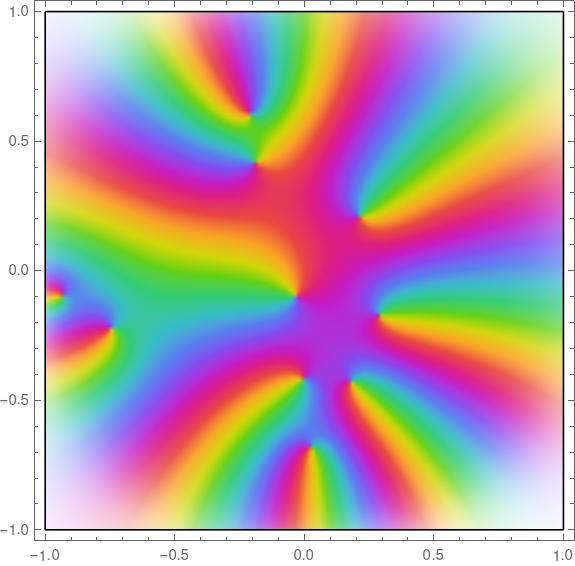Incidentally, if I plot this same function using ComplexPlot3d I get the following.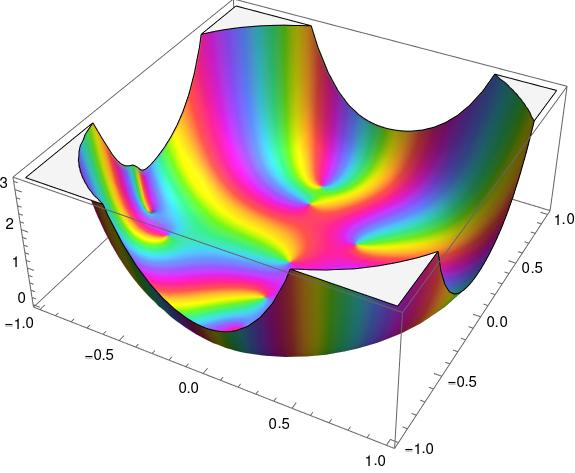Why does it look like a bowl? As explained in the post on Blaschke factors, each factor is zero at its parameter a and has a pole at the reflection of a in the unit disk.

The function plotted above has zeros inside the unit disk near the boundary. That means it also has poles outside the unit disk near the boundary on the other side.

# Inversion in a circle

Inversion in the unit circle is a way of turning the circle inside-out. Everything that was inside the circle goes outside the circle, and everything that was outside the circle comes in.

Not only is the disk turned inside-out, the same thing happens along each ray going out from the origin. Points on that ray that are inside the circle go outside and vice versa. In polar coordinates, the point (r, θ) goes to (1/r, θ).

## Complex numbers

In terms of complex numbers, inversion in the unit circle amounts to taking the reciprocal and the conjugate (in either order, because these operations commute). This is the same as dividing a complex number by the square of its magnitude. Proof:There are two ways to deal with the case z = 0. One is to exclude it, and the other is to say that it maps to the point at infinity. This can be made rigorous by working on the Riemann sphere rather than the complex plane. More on that here.

## Inverting a hyperbola

The other day Alex Kantorovich pointed out on Twitter that “the perfect figure 8 (or infinity) is simply the inversion through a circle of a hyperbola.” We’ll demonstrate this with Mathematica.

What happens to a point on the hyperbolawhen you invert it through a circle? If we think of x and y as the real and imaginary parts of a complex number, the discussion above shows that the point (x, y) goes to the same point divided by its length squared.Let (u, v) be the image of the point (x< y).Then we haveandbecause x² – y² = 1. Notice that the latter is the square of the former, i.e.Now we have everything to make our plot. The code

ContourPlot[{
x^2 + y^2 == 1,
x^2 - y^2 == 1,
x^2 - y^2 == (x^2 + y^2)^2},
{x, -3, 3}, {y, -3, 3}]


produces the following plot.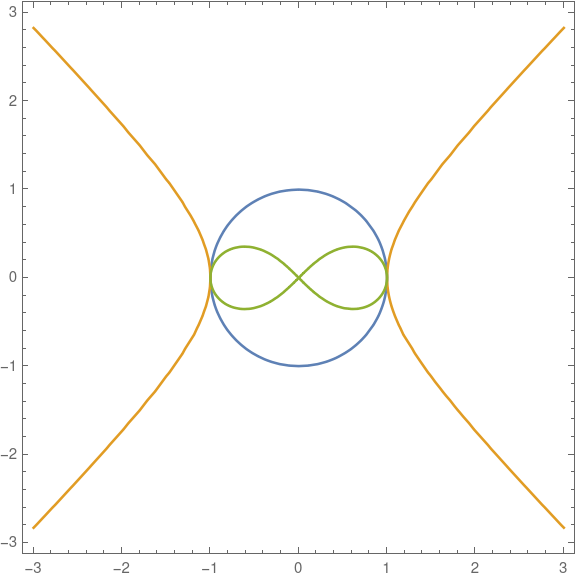The blue circle is the first contour, the orange hyperbola is the second contour, and the green figure eight is the third contour.

# Catenary kiln

Will Buckner sent me an email with the following question recently. (I’m sharing this with permission.)

I am building a kiln using a catenary arch. The rear wall and front wall/door will be vertical and fill in the space under the arch, which has the dimensions of 41″W x 39.5″H. I need the area within this arch in order to calculate how many bricks I need to construct the two walls. Can you help me calculate that area?

Here’s my solution. I fit a parabola and a catenary in part to check my work and in part to see how different they are.

## Parabola

Let w = 41 and h = 39.5. We want a quadratic function y(x) such that y(0) = h and yw/2) = 0.

Assume y has the form

y(x) = ba

y(0) = h leads to the conclusion that b = h, and yw/2) = 0. leads to

a(w/2) = h

And this leads to the final form.

y = h(1 – (2x/w)²)

## Catenary

Our catenary will have the form

y(x) = ba cosh(x/a)

and again y(0) = h and yw/2) = 0. These two requirements lead to the equations

ba = h

and

b – a cosh(w/2a) = 0.

Then

b = h + a

and

h + a (1 – cosh(w/2a) = 0.

The latter equation cannot be solved in closed form, but it’s easy enough to solve numerically. When I asked Mathematica to compute the root with

    FindRoot[39.5 + a - a Cosh[20.5/a] == 0, a]

it failed. Then I plotted the function, saw that the root was around 8.5, then gave Mathematica a hint.

    FindRoot[39.5 + a - a Cosh[20.5/a] == 0, {a, 8.5}]

Then Mathematica came back with

a = 8.475359432102444.

So the equation of our catenary is

y = h + a(1 – cosh(0.5 x/a))

where h and a are given above.

## Plots

Here’s a plot of both curves. The blue curve inside is the parabola, and the gold curve on the outside is the catenary.Here’s the code that made the plot.

    Plot[{h (1 - (2 x/w)^2), h + a - a Cosh[x/a] }, {x, -w/2, w/2}]

## Area

And now for the area, the thing I was asked to find. For the parabola

    Integrate[h (1 - (2 x/w)^2), {x, -w/2, w/2}]

returns 1079.67. For the catenary,

    Integrate[h + a (1 -  Cosh[x/a]), {x, -w/2, w/2}]

returns 1166.56.

The number of bricks this will take is roughly the area of the arch divided by the area of a brick, but being more precise is a complicated question that depends on how much mortar you use, and how you use plan to use rectangular bricks to make a curved arch.

# A closer look at zero spacing

A few days ago I wrote about Kneser’s theorem. This theorem tells whether the differential equation

u ″(x) + h(x) u(x) = 0

will oscillate indefinitely, i.e. whether it will have an infinite number of zeros.

Today’s post will look at another theorem that gives more specific information about the spacing of the zeros.

Kneser’s theorem said that the growth rate of h determines the oscillatory behavior of the solution u. Specifically, if h grows faster than ¼x-2 then oscillations will continue, but otherwise they will eventually stop.

## Zero spacing

A special case of a theorem given in  says that an upper bound on h gives a lower bound on zero spacing, and a lower bound on h gives an upper bound on zero spacing.

Specifically, if h is bound above by M, then the spacing between zeros is no more than π/√M. And if h is bound below by M′, then the spacing between zeros is no less than π/√M′.

Let’s look back a plot from the post on Kneser’s theorem: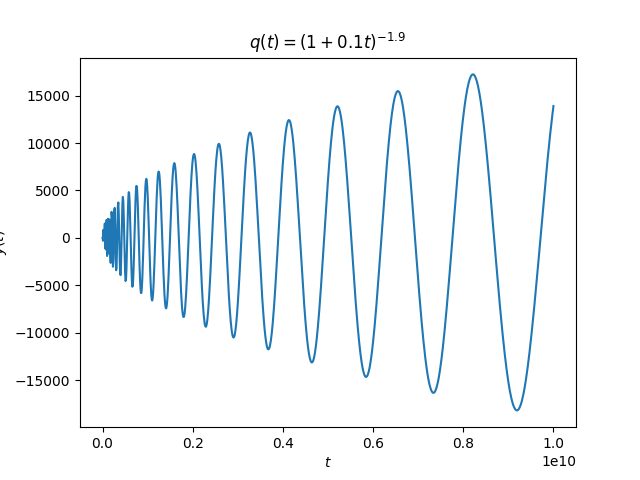The spacing between the oscillations appears to be increasing linearly. We could have predicted that from the theorem in this post. The coefficient of the linear term is roughly on the order of 1/x² and so the spacing between the zeros is going to be on the order of x.

Specifically, in the earlier post we looked at

h(x) = (1 + x/10)p

where p was 1.9 and 2.1, two exponents chosen to be close to either side of the boundary in Kneser’s theorem. That post used q and t rather than h and x, but I changed notation here to match .

Suppose we’ve just seen a zero at x*. Then from that point on h is bounded above by h(x*) and so the distance to the next zero is bounded below by π/√h(x*). That tells you where to start looking for the next zero.

Our function h is bounded below only by 0, and so we can’t apply the theorem above globally. But we could look some finite distance ahead, and thus get a positive lower bound, and that would lead to an upper bound on the location of the next zero. So we could use theory to find an interval to search for our next zero.

## More general theorem

The form of the theorem I quoted from  was a simplification. The full theorem considers the differential equation

u ″(x) + g(xu′(x) +  h(x) u(x) = 0

The more general theorem looks at upper and lower bounds on

h(x) – ½ g′(x) – ¼ g(x)².

but in our case g = 0 and so the hypotheses reduced to bounds on just h.

## Example

Exercise 3 from  says to look at the zeros of solution to

u ″(x) + ½x²  u ′ + (10 + 2x) u(x) = 0.

Here we have

h(x) – ½ g ′(x)- ¼ g(x)² = 10 + 2xx – 1  = 9 + x

and so the lower bound of 9 tells us zeros are spaced at most π/3 apart.

Let’s look at a plot of the solution using Mathematica.

    s = NDSolve[{u''[x] + 0.5 x^2 u'[x] + (10 + 2 x) u[x] == 0,
u == 1, u' == 1 }, u, {x, 0, 5}]
Plot[Evaluate[u[x] /. s], {x, 0, 5}]


This produces the following.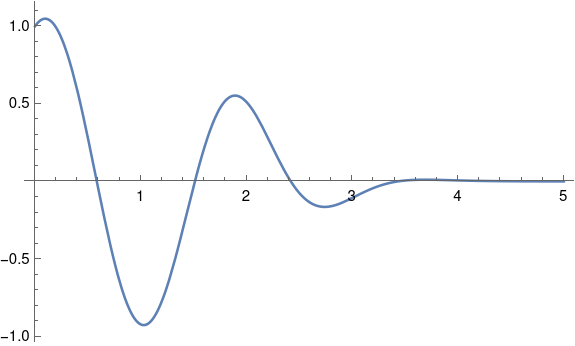There are clearly zeros between 0 and 1, between 1 and 2, and between 2 and 3. It’s hard to see whether there are any more. Let’s see exactly where the roots are that we’re sure of.

    FindRoot[u[x] /. s, {x, 0, 1}]
{x -> 0.584153}

FindRoot[u[x] /. s, {x, 1, 2}]
{x -> 1.51114}

FindRoot[u[x] /. s, {x, 2, 3}]
{x -> 2.41892}


The distance between the first two zeros is 0.926983 and the distance between the second and third zeros is 0.90778. This is consistent with our theorem because π/3 > 1 and the spacing between our zeros is less than 1.

Are there more zeros we can’t see in the plot above? When I asked Mathematica to evaluate

    FindRoot[u[x] /. s, {x, 2, 4}]

it failed with several warning messages. But we know from our theorem that if there is another zero, it has to be less than a distance of π/3 from the last one we found. We can use this information to narrow down where we want Mathematica look.

    FindRoot[u[x] /. s, {x, 2.41892, 2.41892 + Pi/3}]

Now Mathematica says there is indeed another solution.

    {x -> 3.42523}

Is there yet another solution? If so, it can’t be more than a distance π/3 from the one we just found.

    FindRoot[u[x] /. s, {x, 3.42523, 3.42523 + Pi/3}]

This returns 3.42523 again, so Mathematica didn’t find another zero. Assuming Mathematica is correct, this means there cannot be any more zeros.

On the interval [0, 5] the quantity in the theorem is bound above by 14, so an additional zero would need to be at least π/√14 away from the latest one. So if there’s another zero, it’s in the interval [4.26486, 4.47243]. If we ask Mathematica to find a zero in that interval, it fails with warning messages. We can plot the solution over that interval and see that it’s never zero.

 Protter and Weinberger. Maximum Principles in Differential Equations. Springer-Verlag. 1984. Page 46.

# Quintic trinomial root

This post looks at an exercise from Special Functions, exercise 6 in Appendix E.

Suppose that xm+1 + axb = 0. Show thatUse this formula to find a solution to x5 + 4x + 2 = 0 to four decimal places of accuracy. When m = 0 this series reduces to the geometric series. Write this sum as a hypergeometric series.

• It’s a possibly useful result.
• It’s an application of Lagrange’s inversion formula; that’s where the series comes from.
• The equation has m+1 roots. What’s special about the root given by the series?
• The sum is not obviously hypergeometric.
• What happens when the sum diverges? Is it a useful asymptotic series? Does the analytic continuation work?

I want to address the last two points in this post. Maybe I’ll go back and address the others in the future. To simplify things a little, I will assume m = 4.

The parameters of the hypergeometric series are not apparent from the expression above, nor is it even apparent how many parameters you need. It turns out you need m upper parameters and m-1 lower parameters. Since we’re interested in m = 4, that means we have a hypergeometric function of the form 4F3.We can evaluate this expression in Mathematica as

    f[a_, b_] := (b/a) HypergeometricPFQ[
{1/5, 2/5, 3/5, 4/5},
{1/2, 3/4, 5/4},
-3125 b^4 / (256 a^5)
]


When we evaluate f[4, -2] we get -0.492739, which is the only real root of

x5 + 4x + 2 = 0.

Recall that the sign on b is negative, so we call our function with b = -2.

Now lets, try another example, solving for a root of

x5 + 3x – 4 = 0.

If we plug a = 3 and b = 4 into the series at the top of the post, the series diverges immediately, not giving a useful asymptotic series before it diverges.

The series defining our hypergeometric function diverges for |z| > 1, and we’re evaluating it at z = -3125/243. However, the function can be extended beyond the unit disk by analytic continuation, and when we ask Mathematica to numerically evaluate the root with by running

    N{ f[3, 4] ]

we get 1, which is clearly a root of x5 + 3x – 4.

# Numbering the branches of the Lambert W function

The previous post used the Lambert W function to solve an equation that came up in counting partitions of an integer. The first solution found didn’t make sense in context, but another solution, one on a different branch, did. The default branch k = 0 wasn’t what we were after, but the branch k = -1 was.

## Branches 0 and -1

The branch corresponding to k = 0 is the principle branch. In mathematical notation, the k is usually a subscript, i.e W0(z) is the principle branch of the Lambert W function. For real z ≥ -1/e it returns a real value. It’s often what you want, and that’s why it’s the default in software implementations such as SciPy and Mathematica. More on that below.

The only other branch that returns real values for real inputs is W-1 which returns real values for -1/ez < 0.

If you’re working strictly with real numbers, you only need to be concerned with branches 0 and -1. If branch 0 doesn’t give you what you expect, try branch -1, if your argument is negative.

## SciPy and Mathematica

SciPy implements Wk with lambertw(z). The parameter k defaults to 0.

The Mathematica function ProductLog[z] implements W0(z), and ProductLog[k, z] implements Wk.

Note that Mathematica and Python list their arguments in opposite orders.

## Branch numbering

The branches are numbered in order of their imaginary parts. That is, The imaginary part of Wk (z) is an increasing function of k. For example, here is a list of the numerical values of the imaginary parts of Wk (1) for k running from -3 to 3.

    Table[N[Im[ProductLog[k, 1]]], {k, -3, 3}]
{-17.1135, -10.7763, -4.37519, 0., 4.37519, 10.7763, 17.1135}


Note the zero in the middle because W0(1) is real.

## Recovering k

Given z and a value Wk (z) with k unknown, you can determine k withwith the exception that if the expression above is 0 and -1/ez < 0 then k = -1. See .

For example, let z = 1 + 2i and w = W3 (z).

    z = 1 + 2 I
w = ProductLog[3, z]


Now pretend you don’t know how w was computed. When you compute

    N[(w + Log[w] - Log[z])/(2 Pi I)]

the result is 3.

## Partition example

The discussion of the W function here grew out of a problem with partitions. Specifically, we wanted to solve for n such thatis approximately a trillion. We found in the previous post that solutions of the equationare given byOur partition problem corresponds to a = 1/√48, b = -1, c = π √(2/3), and d = 1/2. We found that the solution we were after came from the k = -1 branch.

    N[( (b/(c d)) ProductLog[-1, (x/a)^(d/b) c d /b])^(1/d)]
183.852


Even though our final value was real, the intermediate values were not. The invocation of W-1 in the middle returns a complex value:

    N[ProductLog[-1, ((x/a)^(d/b) c d /b)^(1/d)]]
-32.5568 - 3.24081 I


We weren’t careful about specifying ranges and branch cuts, but just sorta bulldozed our way to a solution. But it worked: it’s easy to verify that 183.852 is the solution we were after.

***

 Unwinding the branches of the Lambert W function by Jeffrey, Hare, and Corless. The Mathematical Scientist 21, 1&ndash;7 (1996)

# Partitions and Pentagons

This post will present a remarkable theorem of Euler which makes a connection between integer partitions and pentagons.

## Partitions

A partition of an integer n is a way of writing n as a sum of positive integers. For example, there are seven unordered partitions of 5:

• 5
• 4 + 1
• 3 + 2
• 3 + 1 + 1
• 2 + 2 + 1
• 2 + 1 + 1 + 1
• 1 + 1 + 1 + 1 + 1

Note that order does not matter. For instance, 4 + 1 and 1 + 4 count as the same partition. You can get a list of partitions of n in Mathematica with the function IntegerPartitions[n]. If we enter IntegerPartitions we get

     {{5}, {4, 1}, {3, 2}, {3, 1, 1}, {2, 2, 1},
{2, 1, 1, 1}, {1, 1, 1, 1, 1}}

We say a partition is distinct if all the numbers in the sum are distinct. For example, these partitions of 5 are distinct

• 5
• 4 + 1
• 3 + 2

but these are not

• 3 + 1 + 1
• 2 + 2 + 1
• 2 + 1 + 1 + 1
• 1 + 1 + 1 + 1 + 1

because each has a repeated number.

We can divide distinct partitions into those having an even number of terms and those having an odd number of terms. In the example above, there is one odd partition, just 5, and two even partitions: 4 + 1 and 3 + 2.

## Pentagonal numbers

The pentagonal numbers are defined by counting the number of dots in a graph of concentric pentagons: 1, 5, 12, 22, …. This is OEIS sequence A000326.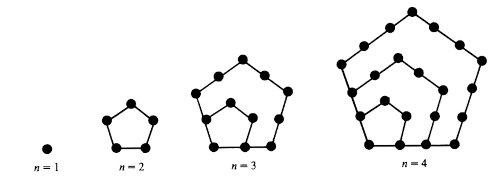The jth pentagonal number is

Pj = j(3j – 1) / 2.

We can define negative pentagonal numbers by plugging negative values of j into the equation above, though these numbers don’t have the same geometric interpretation. (I think they do have a geometric interpretation, but I forget what it is.)

## Euler’s pentagonal number theorem

Leonard Euler discovered that the number of even distinct partitions of n equals the number of odd distinct partitions, unless n is a pentagonal number (including negative indices). If n is the jth pentagonal number, then the difference between the number of even and odd distinct partitions of n equals (-1)j. In symbols,Here De is the number of even distinct partitions and Do is the number of odd distinct partitions.

Euler discovered this by playing around with power series and noticing that the series involved were generating functions for pentagonal numbers. Jordan Bell has written a commentary on Euler’s work and posted it on arXiv.

## Mathematica examples

Let’s look at the partitions of 12, the 3rd pentagonal number.

IntegerPartions shows there are 77 partitions of 12. We can pick out just the partitions into distinct numbers by selecting partition sets that have no repeated terms, i.e. that have as many elements as their union.

    Select[IntegerPartitions, Length[Union[#]] == Length[#] &]

This returns a list of 15 partitions:

    {{12},      {11, 1},   {10, 2},      {9, 3},    {9, 2, 1},
{8, 4},    {8, 3, 1}, {7, 5},       {7, 4, 1}, {7, 3, 2},
{6, 5, 1}, {6, 4, 2}, {6, 3, 2, 1}, {5, 4, 3}, {5, 4, 2, 1}}


Of these sets, 7 have an even number of elements and 8 have an odd number of elements. This matches what Euler said we would get because

7 – 8 = (-1)3.

We’d like to eplore this further without having to count partitions by hand, so let’s define a helper function.

    f[list_] := Module[
{n = Length[Union[list]]},
If [n == Length[list], (-1)^n, 0]
]


This function returns 0 for lists that have repeated elements. For sets with no repeated elements, it returns 1 for sets with an even number of elements and -1 for sets with an odd number of elements. If we apply this function to the list of partitions of n and take the sum, we get

De(n) – Do(n).

Now

    Total[Map[f, IntegerPartitions]]

returns -1. And we can verify that it returns (-1)j for the jth pentagonal number. The code

    Table[Total[Map[f, IntegerPartitions[j (3 j - 1)/2]]], {j, -3, 3}]

returns

    {-1, 1, -1, 1, -1, 1, -1}

We can compute De(n) – Do(n) for n = 1, 2, 3, …, 12 with

    Table[{n, Total[Map[f, IntegerPartitions[n]]]}, {n, 1, 12}]

and get

    {{1, -1}, {2, -1}, {3,  0}, {4,  0},
{5,  1}, {6,  0}, {7,  1}, {8,  0},
{9,  0}, {10, 0}, {11, 0}, {12, -1}}

which shows that we get 0 unless n is a pentagonal number.

## Euler characteristic

Euler’s pentagonal number theorem reminds me of Euler characteristic. Maybe there’s a historical connection there, or maybe someone could formalize a connection even if Euler didn’t make the connection himself. In its most familiar form, the Euler characteristic of a polyhedron is

VE + F

where V is the number of vertices, E is the number of edges, and F is the number of faces. Notice that this is an alternating sum counting o-dimensional things (vertices), 1-dimensional things (edges), and 2-dimensional things (faces). This is extended to higher dimensions, defining the Euler characteristic to be the alternating sum of dimensions of homology groups.

We can write De(n) – Do(n) to make it look more like the Euler characteristic, using Dk(n) to denote the number of distinct partitions of size k.

D0(n) – D1(n) + D2(n) – …

I suppose there’s some way to formulate Euler’s pentagonal number theorem in terms of homology.

# Everywhere chaotic map on the sphere

Let f be a complex-valued function of a complex variable. The Julia set of f is the set of points where f is chaotic. Julia sets are often complicated fractals, but they can be simple. In this post I want to look at the function

f(z) = (z² + 1)² / 4z(z² – 1).

I learned about this function from the latest 3Blue1Brown video. This function is a Lattès example. (I misread this at first and thought it was Latte’s example, but it is one of a family of examples by the French mathematician Samuel Lattès.)

What makes this function interesting is that its Julia set is the entire plane. That is, iterates of the function are sensitive to starting point no matter where you start.

I wanted to play around with this function, but plotting iterates would not be practical if they wander all over the complex plane: no matter how big a region I plot, the points will leave that region, and possibly leave quickly. So instead of looking at iterates on the complex plane, I’ll look project them onto a sphere so we can see them all at once.

## Riemann sphere

This is a good time to come clean about a detail I left out. From the beginning I should have said that f is a map not from the complex plane ℂ to itself but from the Riemann sphere

ℂ ∪ {∞}

to itself. I didn’t gloss over much, just one point, the point at infinity. In our example, we’ll define f(0) = ∞ and the resulting extension is continuous as a map from the sphere to itself.

Not only will moving to the sphere make things easier to see, it’s actually where our function naturally lives.

## Stereographic projection

Imagine a unit sphere sitting on top of the complex plane. Stereographic projection maps every point on the sphere, except the north pole, to a point in the plane. Draw a line between the north pole and a point on the sphere, and its stereographic projection is where the line intersects the plane. The north pole itself has nowhere to go. When we extend the complex plane by adding a point ∞, we can map the north pole there.

We’re actually interested in the inverse of stereographic projection because we want to go from the plane to the sphere. Let’s define a function p[u, v] that carries out our inverse stereographic projection in Mathematica.

    p[u_, v_] := ResourceFunction[
"InverseStereographicProjection"][{u, v}]

## Plotting iterates

We want to pick some arbitrary starting point z0 and look at the sequence

z0, f(z0), f(f(z0)), f(f(f(z0))), …

We can do this in Mathematica with the NestList function. It takes three arguments: the function to iterate, a starting point, and the number of elements in the sequence to produce. For example, if we define

    latte[z_] := (z^2 + 1)^2/(4 z (z^2 - 1))

then

    NestList[latte, 0.1 + 2 I, 5]

gives us the first five elements in the sequence above.

The projection function p[u, v] above takes x and y coordinates, not complex numbers, so we define our own that takes complex numbers.

    projection[z_] := p[Re[z], Im[z]]

Now we’re can plot our iterates. I chose 10 + 1.9i as a starting point based on today’s date, October 19, but you could pick any non-zero starting point. And that’s kinda the point: any place you start will have the same chaotic dynamics .

Here’s our plotting code.

    ListPointPlot3D[
Map[projection, NestList[latte, 10 + 1.9 I, 1000]],
AspectRatio -> 1]


And here’s the plot: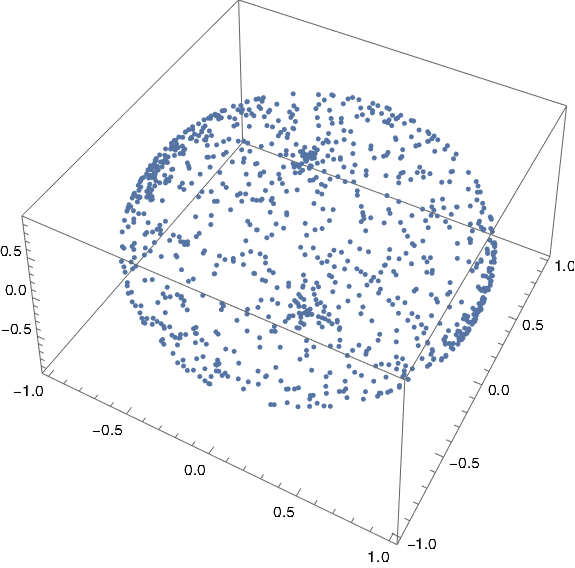We could plot a sphere with

    SphericalPlot3D[1, {t, 0, Pi}, {ph, 0, 2 Pi}]

and stack the two plots to make it clear that the points are indeed on a sphere.## Related posts

 If you start with 0, you’ll next go to ∞ and stay there. But 0 and ∞ are parts of the Julia set too because the points in the neighborhoods of these points lead to chaos, no matter how small you take the open neighborhoods to be.

# Plotting the Gauss map

A recent post looked at an example from one of Michael Trott’s tomes. This post looks at another example from the same tome.

Trott made a contour plot of the Gauss mapover the complex plane. I copied his code (almost) and reproduced his plot.

    ContourPlot[
Abs[1/(x + I y) - Floor[1/(x + I y)]],
{x, -1.1, 1.1}, {y, -1.1, 1.1},
PlotPoints -> 40, ColorFunction -> Hue,
ContourStyle -> {Thickness[0.00001]}
]


This produced the following image.The original code set PlotPoints to 400, and it was taking forever. I got impatient and set the parameter to 40. Looking at the image in the book, it seems the plot with the parameter set to 400 is very similar, except there’s a black tangle in the middle rather than a white space.

In 2004 when Trott wrote his book, Mathematica did not have a command for plotting functions of a complex variable directly, and so Trott rolled his own as a function of two real variables.

Here’s a plot of the same function using the relatively new ComplexPlot function.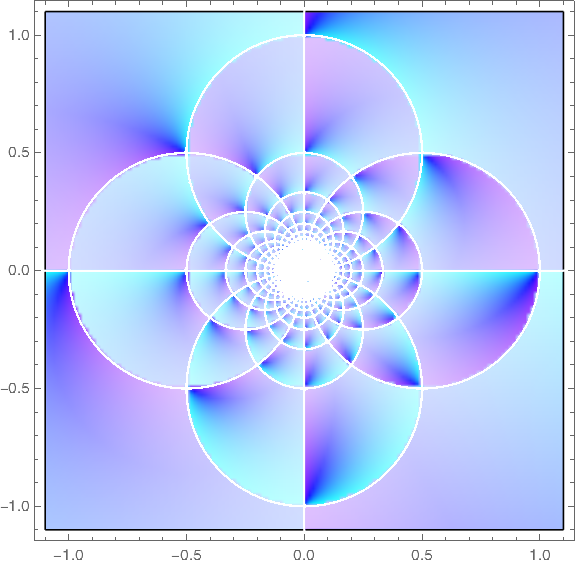Here’s the code that produced the plot.

    ComplexPlot[
(1/z - Floor[1/z]),
{z, -1.1 - 1.1 I, 1.1 + 1.1 I},
ColorFunction -> Hue, PlotPoints -> 400
]


## Update

What does it mean to apply the floor function to a complex number? See the next post.

The plots above were based on Mathematica’s definition of complex floor. Another definition gives different plots.

    aplfloor[z_] :=
With[ {x = Re[z] - Floor[Re[z]], y = Im[z] - Floor[Im[z]], g = Floor[z]},
If[x + y > 1, If[x >= y, g + 1, g + I], g]]


This leads to different plots of the Gauss map.

Vintage: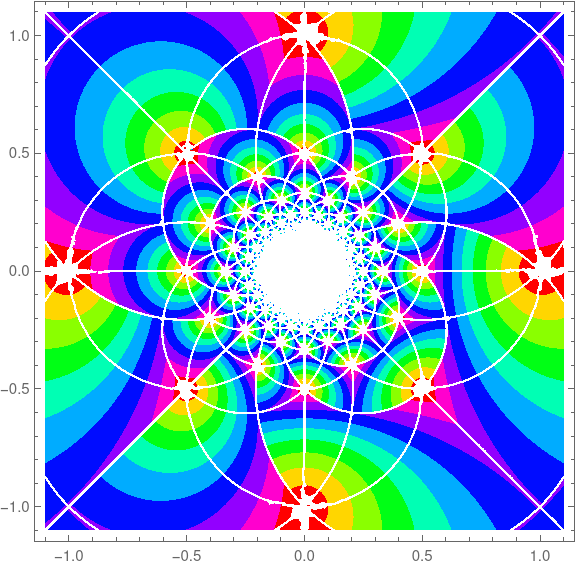And new style: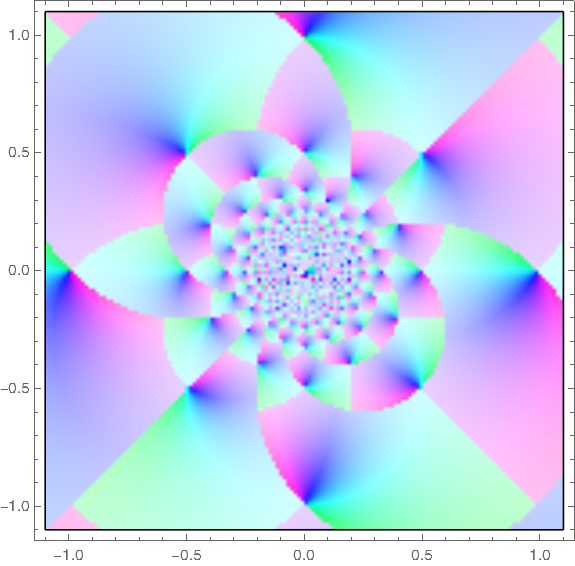# Nonlinear phase portrait

I was reading through Michael Trott’s Mathematica Guidebook for Programming and ran across the following plot.I find the image aesthetically interesting. I also find it interesting that the image is the phase portrait of a differential equation whose solution doesn’t look that interesting. That is, the plot of (x(t), x ‘(t)) is much more visually interesting than the plot of x(t).

The differential equation whose phase portrait is plotted above iswith initial position 1 and initial velocity 0. It’s a familiar damped, driven harmonic oscillator, except the equation is nonlinear because the derivative term is cubed.

Here’s a plot of the solution as a function of time.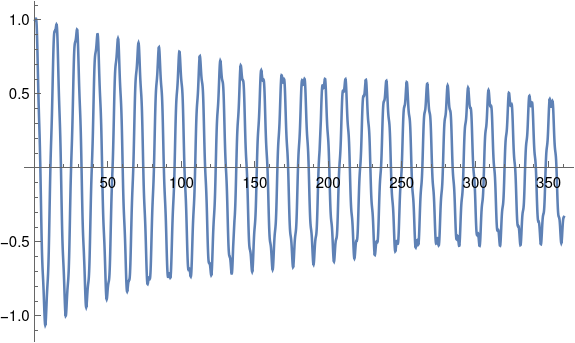The solution basically looks like the solution of the linear case, except the solutions are more jagged near the local maxima and minima. In fact, the plot looks so jagged that I wondered whether this was an artifact of needing more plotting points. But adding more points didn’t make much difference. Interestingly, although this plot looks jagged, the phase portrait is smooth.

I produced the phase portrait by copying Trott’s code and making a couple small modifications.

    sol = NDSolve[{(*differential equation*)
x''[t] + 1/20 x'[t]^3 + 1/5 x[t] ==
1/3 Cos[E t],(*initial conditions*)x == 1, x' == 0},
x, {t, 0, 360}, MaxSteps -> Infinity]

ParametricPlot[Evaluate[{x[t], x'[t]} /. sol], {t, 0, 360},
Frame -> True, Axes -> False, PlotPoints -> 3600]


Apparently the syntax of NDSolve has changed slightly since Trott’s book was published in 2004. The argument x in the code above was written x[t] in Trott’s original code and this did not work in the current version of Mathematica. I also simplified the call to ParametricPlot slightly, though the original code would work.